GNSS Geodesy Innovation Interviews UAV/UAS Positioning Surveying Applications Perspective Time Navigation Mapping Policy Land Administration News Archives LBS GIS SDI Imaging E-ZinePositioning

# Positional accuracy enhancement of an INS/DGPS integrated system

Sep 2006 | Comments Off on Positional accuracy enhancement of an INS/DGPS integrated system

###### This article presents a novel cascade denoising algorithm to reduce the impact of short term INS errors and improve the positioning accuracy during GPS signal blockages using several INS/DGPS integrated navigation systems

According to Skaloud , the inertial sensor errors are composed of long term errors (low frequency components) and short term errors (high frequency components). Therefore, a conceptual plot of the frequency spectrum of the inertial sensor errors in the measurements can be illustrated as in Figure (1).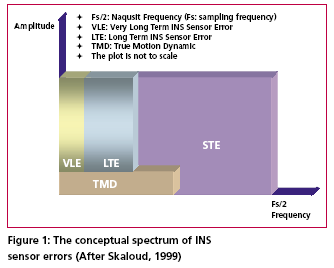Figure (2) shows how each of the errors is reduced by the INS/DGPS integration process. The long term errors are reduced by updating the filter with the observed error state vector coming from the GPS filter (position and velocity). Certain amounts of the short term errors are reduced by the smoothing that is done by the numerical integration process of the INS mechanization [Burton et al., 1999]. However, Figure (2) indicates the benefits of the INS/ DGPS integration are band-limited as the lower boundary of the error spectrum is mainly determined by remaining biases in the GPS observations while the upper boundary is mainly determined by short term inertial sensor errors.Consequently, the remaining GPS biases contained within the GPS navigation solution, such as ionospheric delay, tropospheric delay and multipath, are responsible for the very long term errors illustrated in Figures (1) and (2). Due to restrictions set forth by the sampling theory, the utilization of DGPS data to reduce the short term INS errors is not effective since the sampling rate of DGPS measurements (1Hz) is much lower than those of an inertial unit. As a result, the long term INS errors that are reduced by the integration process with GPS are usually more significant than the short term errors [Skaloud, 1999].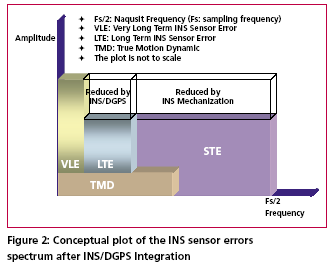The long term errors usually include accelerometer biases and gyro drifts which are commonly modeled as error states. Therefore, the impact of these long term errors for long periods of time can be limited with external aiding. On the contrary, the remaining short term errors remain and contribute to the error accumulation during GPS signal outage periods. Consequently, Figure (3) illustrates that a perfect denoising algorithm is expected to preserve the true motion dynamic signal and remove unwanted short term errors completely. In addition, Figure (3) also implies that the key element of developing the pre-filtering algorithm for removing unwanted short term errors is to investigate the bandwidth of the true motion dynamics sensed by each inertial sensor individually. Thus, the objectives of this article are to: (1) identify the motion dynamic bandwidth of a typical land vehicle sensed by each inertial sensor individually, (2) evaluate the performance of the proposed algorithm using various INS/ DGPS integrated land vehicle systems, and (3) investigate the negative impacts of the cascade denoising algorithm on different INS/DGPS integrated land vehicle systems.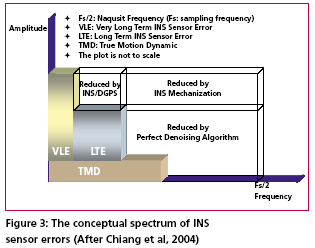## Spectrum analysis of IMU kinematic signals

high frequency (short term) error components of IMU signals. See Skaloud , Burton et al., , and Nassar  for details. However, for land vehicle navigation applications the concern is in removing short term errors and improving the positioning accuracy during GPS signal blockages without jeopardizing the true motion dynamic components of the vehicle.

Indeed, such operation requires the prior knowledge of the true bandwidth for typical land vehicle motion dynamics and the spectrum characteristics of the wavelet denoising algorithm. Thus, the positioning errors, after applying denoised kinematic IMU measurements, can be expected to be smaller than those obtained through the use of original data if the true motion dynamic content can be well preserved and the short term errors can be removed during the denoising operation. Chiang et al.,  investigated the bandwidth of true motion dynamics using kinematic IMU raw measurements sensed by several systems and suggested the bandwidth of true motion dynamics for general land vehicle applications as given in Table 1. See Chiang  and Chiang et al.,  for more details about the spectrum analysis of kinematic IMU signals. The cause for the wider bandwidth sensed by the X-Gyro, YGyro YGyro and ZAccelerometer was mainly due to road irregularities (i.e., bumps). In contrast, the narrower bandwidth sensed by the XAccelerometer, YAccelerometer and Z-Gyro indicates a much smoother heading motion along the trajectory, which reflects the dynamic motion variation in typical land vehicle applications [Czonpo, 1990, Chiang et al., 2004 and Chiang 2004].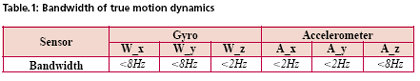Mallat  proposed Multiresolution Analysis (MRA), which has been the most common and general approach to constructing a wavelet basis. In signal processing, such an idea is implemented as subband filtering, or quadrature mirror filtering [Strang and Nguyun, 1997]. The decomposition step consists of a low pass (h) and a high pass (g) filter followed by downsampling (? 2) (i.e., retaining only the even index samples), see Mallat  for more details about wavelet decomposition. Chiang et al.,  investigated the relationship between the decomposition level, sampling frequencies (i.e., Fs=200Hz, Fs=100Hz, and Fs=50Hz), and the stop bands of residual frequencies corresponding to the approximate signals through the spectrum analysis of approximation signals (Ai,i=1, 2, 3…n) and the detail signals (Di,i=1, 2, 3…n) generated at each wavelet decomposition level, as indicated in Table 2.

The relationship presented in Table 2 was derived using Daubechies wavelet functions (DB(i), i=2
~ 15) [ Daubechies, 1992].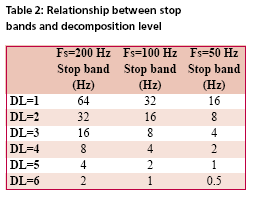### First Generation Wavelet Denoising Algorithm

The basic principle of the first generation denoising algorithm is to perform thresholding on the DWT of the noisy signal, and then take the inverse DWT of the thresholded coefficients to obtain the denoised signal. Donoho  proposed the following schemes for denoising:

1) Suppose x(n) is the original signal of length n, y(n) = x(n) + e(n), where y(n) is corrupted by e(n) ~ N (0,1). Find the DWT of y(n) which is called Yj, k(n).

2) Perform proper thresholding on Yj, k(n) using d chosen based on Stein’s Unbiased Estimate of Risk or Threshold choice (SURE) (see Donoho  for details) , see Chiang et al.,  for details about performance of different thresholding algorithms.

3) Take the inverse DWT of k j X , ˆ to
recover the denoised signal ˆ ( ) X L

### Second Generation Wavelet Denoising Algorithm

The DWT is not translation invariant (shift invariant), meaning that if a DWT is applied to a shifted version of a signal x, it cannot get the shifted version of the DWT of x [Lang et al, 1996]. The lack of translation invariance is not necessarily a problem for most applications, but for denoising this phenomenon introduces artifacts when using transform domain thresholding dependent on the kind of transform domain one is working in [Jansen, 2001]. For wavelet denoising the artifacts are related to the behavior near singularities. In the neighborhood of discontinuities, wavelet denoising can exhibit pseudo-Gibbs phenomena [Coifman and Donoho, 1995].To reduce the impact of pseudo-Gibbs phenomena, the Undecimated Wavelet Transform (UDWT), which has been independently discovered under several names, e.g., shift/translation invariant wavelet transform (TIW) [Coifman and Donoho, 1996], stationary wavelet transform (SWT) [Nason and Silverman,1995] or redundant wavelet transform, can be applied.

Coifman and Donoho  extensively studied the similar characteristics of the UDWT and implemented a so called Translation Invariant Wavelet Transform (TIW) based on the idea of Cycle-Spinning, or denoising all possible shifts of a signal and then averaging. The idea was originally explored to reduce the pseudo-Gibbs phenomena. If we let Sh represent the circular shift operator then for a signal X with length N, ShX(k) = X((k+h) mod N). Now if L represents the DWT operator, T represents the thresholding operator, Sh -1 and L-1 are the unshift and IDWT operators respectively, then the denoised signal is given by the following equation: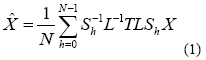The denoising procedure for IMU raw measurements is illustrated in Figure (4) where cAi (i = 1, 2, 3…n) and cDi (i = 1, 2, 3…n) are approximation and detail coefficients generated at each decomposition level. See Chiang  for the relationship between approximation/detail signals and approximation/detail coefficients. The optimal decomposition level (L) varies with the bandwidth of true motion dynamics in each sensor. It can be chosen using Table 2 and the bandwidth of the true land vehicle motion dynamics listed in Table 1, which is given in Table 3.

Chiang et al.,  indicated that the major limitation of applying either the 1st or 2nd generation denoising algorithms was the remaining high frequency components. The conceptual plot of the frequency spectrum of both the 1st and 2nd generation denoising algorithms is given in Figure (5). Therefore, the remaining issue involves removing the short term errors whose frequencies are higher than the stop band and reducing the short term errors whose frequencies are lower than the stop band when the existing wavelet denoising algorithm is applied to the IMU signals.

«Previous 1 2View All| Next»

Pages: 1 2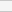(No Ratings Yet)Loading...